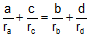# Online Geometry Problem 447: Complete Quadrilateral, Inradii, Exradii, Sides

 The figure shows a complete quadrilateral ABCDEF. If ra, and rd are the exradii of triangles ABE and ADF, and rb, and rc are the inradii of triangles BCF and CDE, prove that. Complete quadrilateral ABCDEF is the figure determined by four lines, no three of which are concurrent, and their six points of intersection A, B, C, D, E, and F. Exradius is the radius of an excircle. Excircle is the circle tangent to the extension of two sides and to the other side, Inradius is the radius of an incircle. Incircle is the circle tangent to each of the triangle's three sides.Home | Search | Geometry | Problems | All Problems | Visual Index | Complete Quadrilateral | 441-450 | Email | Solution / comment | By Antonio Gutierrez